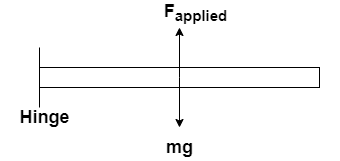# Problem: A uniform 300-N trapdoor in a ceiling is hinged at one side.a) Find the net upward force needed to begin to open it, if the upward force is applied at the center.b) Find the total force exerted on the door by the hinges, if the upward force is applied at the center.

###### FREE Expert Solution

Torque:

$\overline{){\mathbf{\tau }}{\mathbf{=}}{\mathbf{r}}{\mathbf{F}}{\mathbf{s}}{\mathbf{i}}{\mathbf{n}}{\mathbf{\theta }}}$

Newton's second law:

$\overline{){\mathbf{\Sigma F}}{\mathbf{=}}{\mathbf{ma}}}$

Let the length of the door be L.(a)

The weight and the applied force are at a distance L/2

The forces and radial distance are perpendicular — θ = 90°

ΣT = 0

(L/2)•mgsin 90 - (L/2)Fapplied sin 90 = 0

98% (204 ratings)###### Problem Details

A uniform 300-N trapdoor in a ceiling is hinged at one side.

a) Find the net upward force needed to begin to open it, if the upward force is applied at the center.

b) Find the total force exerted on the door by the hinges, if the upward force is applied at the center.